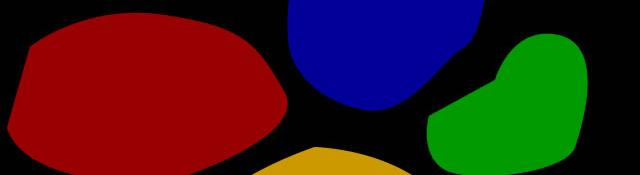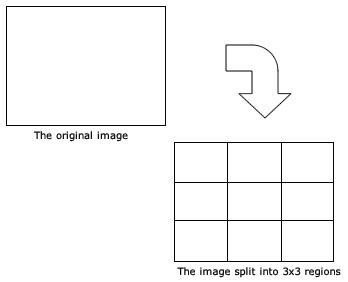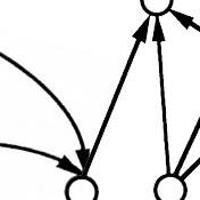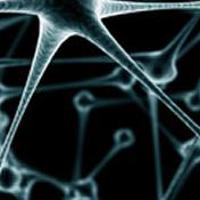## Fast connected components labeling

Labeling connected components in an image is a common operation. But the original algorithm proposed is slow. It works fine if the image is small. But as the image becomes larger, the algorithm slows down really fast. Recently, a few researchers find a simple solution to this. Image that previously took hundreds of seconds would not take only a couple of seconds to get labeled.

## The original connected components labeling algorithm

A binary image has only ones and zeroes. The goal of labeling connected components is to identify which ones are "connected".For example, if the above image, the goal is to identify that the two circles on the left are connected. Sure, they are separate circles, but they are "connected". They share the same boundary. Similarly for the two circles on the right.

The original algorithm is a two pass algorithm. The first pass marks labels for each pixel and the second pixel marks corrects invalid labels. You might want to read more about the connected components labeling algorithm. You might also want to check an example on how the connected components labeling algorithm works.

## The divide and conquer approach

The main bottleneck of the algorithm is the large equivalence array (the union-find structure). Calculating and resolving equivalent labels for one big image takes a lot of time. However, if the image is divided into several regions (say the image is split into 3x3 parts), then the labels can be computed and resolved much faster.After splitting, you execute the standard algorithm on each part. Now you need to resolve any anomalies that might exist at the borders of each part.

This resolution is done in three parts:

1. Check the top-left pixel of each part (this pixel connects three other parts: to the left, above and the one diagonally)
2. Check the leftmost column of pixels (this column connects the current part to the left)
3. Check the topmost column of pixels (this column connects the current part to the part above it)

For each case, you fix any wrong labeling that might exist.

The next step is to determine the number of pieces the image is split in. Experimentally, it was found that each piece should be 3030 pixels - 6060 pixels in size. That way, the equivalence array is small enough to be computed rapidly.

## Results

The researchers did experiments with a 1760*1168 image. This image is HUGE. The standard algorithm would take a lot of time. It simply isn't feasible to calculate the time it takes.

On dividing, the following times were obtained:

• 4x4 split: 274.57 seconds
• 5x5 split: 160.22 seconds
• 10x10 split: 12.47 seconds
• 15x15 split: 4.01 seconds
• 20x20 split: 2.75 seconds
• 25x25 split: 2.47 seconds

They also compared it against other methods of computing labels. This algorithm clearly surpassed the results any other algorithm could produce.

## Summary

You learned about a new connected component labeling algorithm that computes labels for large images really fast. Have a look at the actual paper for pseudo code on how to implement it.

#### Related posts#### 7 unique neural network architectures